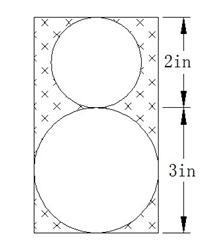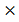### Sample Problem

The figure is made up of a rectangle with two circles cut out. What is the area of the shaded part of the figure? Take π = 3.14 and round your answer to two decimal places.

in2#### Solution

Area of the rectangle = (2 + 3)3 = 15 in2.

Radius of the bigger circle = 3/2 = 1.5 in.

Radius of the smaller circle = 2/2 = 1 in.

Area of the bigger circle = 3.141.51.5 = 7 in2.

Area of the smaller circle = 3.1411 = 3.14 in2.

The area of the shaded part of the figure = 15 - 7 - 3.14 = 4.86 in2.

So, the area of the shaded part of the figure is 4.86 in2.